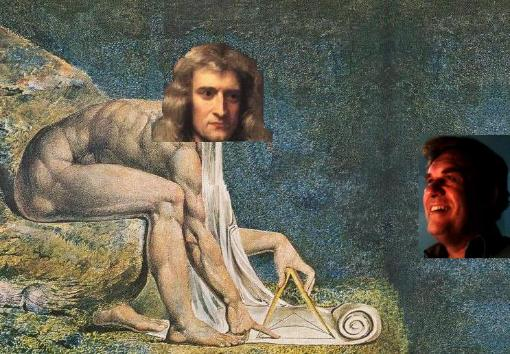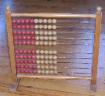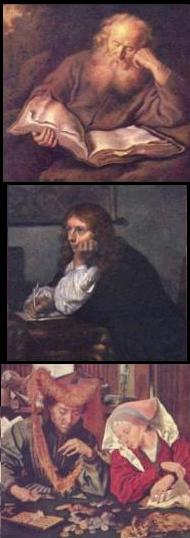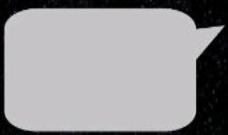Adapted for the Internet from:Why God Doesn't Exist

1.0   What a number is not

The mathematicians have gotten so involved with their idiotic discipline, certainly far beyond any justification, that they utter
the most stunning remarks. Spector claims that a number, for example the number 5, is a physical object. He says that a number
is like a chair or like a rock because he can write them on paper:

" Natural numbers are physical, whether material or conceptual. Numerals obviously
are physical, they are written on paper, and we calculate with them according to
their written appearance on the page.  Mathematics at every level is concerned with
physical form." 

Material numbers? What is it that the mathematicians smoke? What are these people into?

Of course, it all starts when the mathematicians don't define the crucial words that underlie their theories and sweeping
conclusions. If we define physical and material as 'that which has shape,' the number 5 obviously does not qualify as 'physical.'
This is the only manner to define the word physical in order to use it consistently (i.e., scientifically).

The devil's advocate may argue that the number 5 has shape. After all, we can all see a shape in front of our eyes.

However, this argument is easily demolished. First of all, the proponent is confusing form with pattern. A chair has shape. A 5
is a pattern: an incomplete shape.

Nevertheless, the number 5 is a symbol that represents how many times the mathematician moved his hand up and down to
count kangaroo jumps. The number 5 has no significance without its relation to other numbers. The mathematician cannot use
'shape' in the context of calculations. The symbol 5 has relevance only for its conceptual value. The 'object' 5 (i.e., its shape) is
irrelevant. Spector at least agrees on that much:

" The symbol '5' or 'V' is not a number, any more than the word  'cat' will ever
say 'meeow'. " 

For instance, if a mathematician points to a 5, the ET would visualize a shape, but not understand the meaning of this 'thing.'
The word five does not refer the S-shape. The word five refers to the abstract value. This is what has meaning to the
mathematician and not its shape. Indeed, the shape is incidental. For instance, the Romans used the letter V to designate a five.
We can use any symbol we want because the shape of the symbol is not what is important. The concept five is simply not a
physical object. It does not have shape. What has shape is the petty symbol used to designate the value. The mathematicians
have no use for the object 5. The object 5 does not exist in the real world any more than God or leprechauns exist in the real
world.

The mathematicians have a hard time distinguishing between objects and concepts. They can't seem to tell the difference
between an object such as the heart and a concept such as love, or between the elephant and its  location. As a number, 5 is
merely a relation: a concept.  When Spector says that 5 is 'physical' he is using inconsistent language (i.e., relying on an
unscientific definition). Spector is using ordinary speech to draw sweeping scientific conclusions. Spector is clearly a
mathematician. I don't know this because he has a degree from some university. I know this because of the ridiculous
definitions and outrageous conclusions he comes up with.

Of course, Spector is welcome to define unambiguously what he means by 'physical' and 'material' if he disagrees with my
assessment.

2.0   Counting is not the same as size

Some mathematicians perhaps think about magnitudes when they use the word number and, thus, confuse a number with a
size.

" There's not much one can say specifically about the number zero. It's just like any
other whole number: it's an abstract concept measuring the size of a set. The number
zero measures the size of the set with no elements in it, just like the number one
measures the size of the set with a unique element in it, and so on." 

The mathematicians have not learned the fundamental notions about their own field of expertise. A number is not a 'size.'
Size is something you measure. You need another object or standard to compare 'size.' A number, on the other hand, is the
result of something you counted.

It is the mathematicians' insistence on using unscientific language and confusing measurement with counting that leads them
to their ridiculous conclusions. It is irrational in Science to say that 5 is the 'size' of a set. Would it make sense to say that 5 is
the size of a mathematician's ignorance? What the mathematician is trying to say in his clumsy way is that the set contains 5
items. This is not its size (ordinary speech, unscientific terminology). This is how many items we counted. An elephant may
have size, and it certainly will not just be a number. The number will be accompanied by a unit, for example, feet or meters.

3.0   So what is a number?

When asked to define a word, some of these college boys want to be as scientific as possible and make it much more
complicated than it really is. Predictably, they end up with an incorrect definition.

Take the word number. The 'high-level' Math site at Wolfram defines it as:

" A concept of quantity, usually a collection of units." 

" The word 'number' is a general term which refers to a member of a  given (possibly
ordered) set. The meaning of 'number' is often clear from context" 

[If we can infer a word's meaning from the context, why take the trouble to define
anything?]

But is this correct? Is a number 'a member of a set'? A fellow is white, anglo-saxon, and protestant. He belongs to the set of
WASPs. Does he qualify as a number? How about an apple and a gay man? Do they belong to the set of numbers known as
fruits? And then we have the verbs run, swim, and jump. Are they numbers? In their extreme eagerness to come across as
scientific and formal as they possibly can, the mathematicians at Wolfram end up looking like fools!

Other sources have more down to earth definitions that everyone can relate to and which are more correct.

" A number is an abstract entity that represents a count or measurement." 

[The 'measurement part is incorrect. A number is NOT a measurement!]

" a word or symbol, or a combination of words or symbols, used in counting or in
noting a total." 

A number is the verb 'to count'. This is all it is! The mathematicians have converted this verb into a noun. End of story!

Therefore, it is misleading to say that a number is 'an entity'. This leads some to confuse the shape of the symbol '5' with a
physical object. It isn't. This further leads them to believe that they can move the 5 around.

" First, carry down the '2' that indicates the leading coefficient...Multiply by the number
on the left, and carry the result into the next column" 

For the purposes of Science, a number is a concept. A number is a relation. We cannot understand 5 without 1 or 4 or 6.
Thus, a number is more appropriately defined as an adverb. If counting is what a number is, a specific number tells us how
many times we must move our hands up and down to count something. The mathematicians have assigned symbols such
as 1 or 5 or 10 to distinguish these iterations. The number 5 does not say anything about the structure of a chair or of a rock.
The number 5 is a qualifier that tells you that you must move your hand up and down one more time than 4 and one less
than 6. The number 5 is a symbol that tells you HOW far you've counted. For the purposes of Science, all 'hows' are classified

Therefore, it is okay to treat the word number (or the number 5) as a noun for the purposes of ordinary speech. It is not okay
to do this in Science. Mathematics is the discipline that deals with numbers. Mathematics is a discipline that deals exclusively

My argument may seem reasonable and straight forward to some mathematicians. So what?

The problem comes when the idiots of Mathematics believe that numbers exist or that zero is a number. The problem comes
when they believe that there are such things as negative numbers or that infinity is a number and propagate this nonsense
from generation to generation. Then, the mathematicians unilaterally decree that numbers constitute the language of Physics,
but wonder why they can't answer simple questions asked by a child.
 What is a number?
 The three 'R' verbs of civilization:Reading   Riting   and   Rithmeticwriting
 countingI understand what reading means. I understand what writing means. But I don't understand what numbers are.I wouldn't worry too much about that right now if I were you,Newt. You will soon understand that this is not a nudist beach and that there are a couple of cops behind you.________________________________________________________________________________________